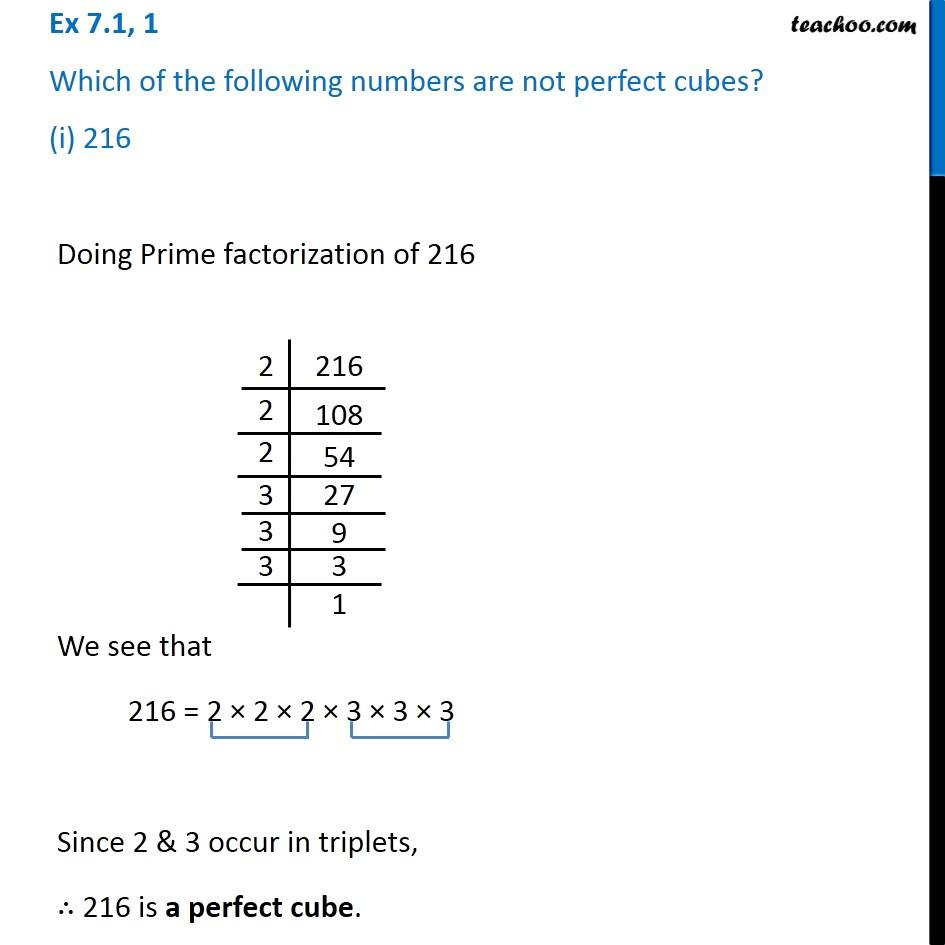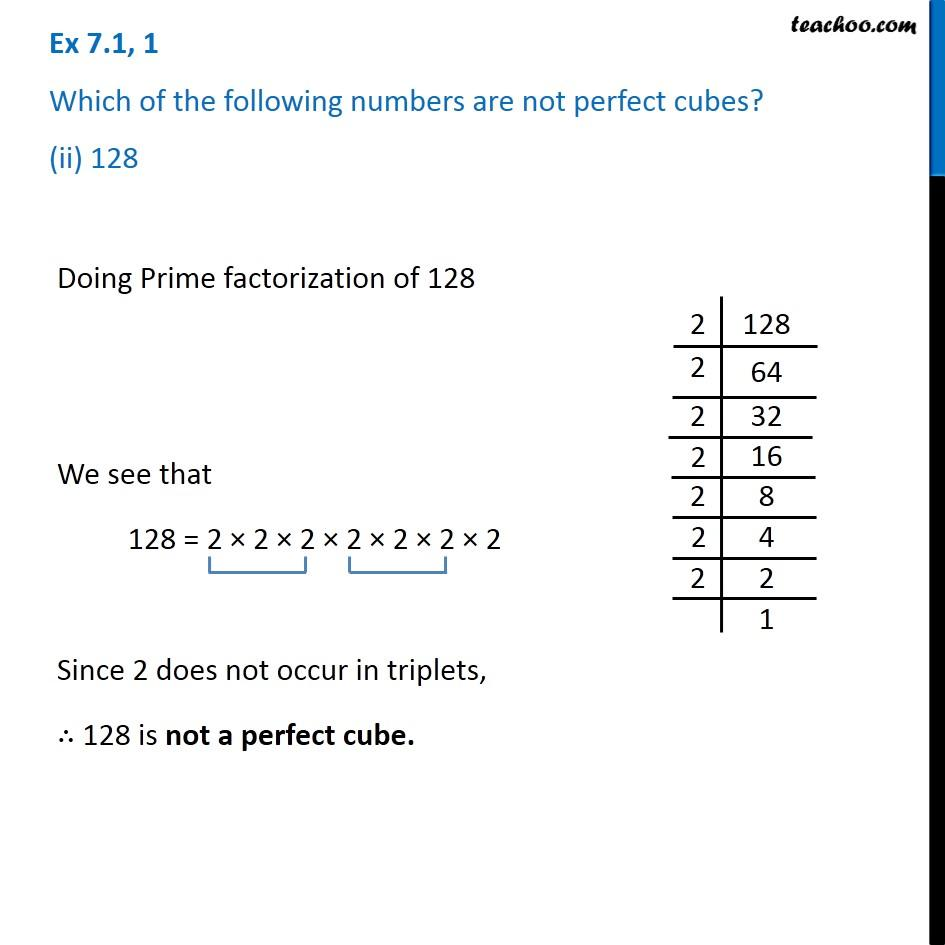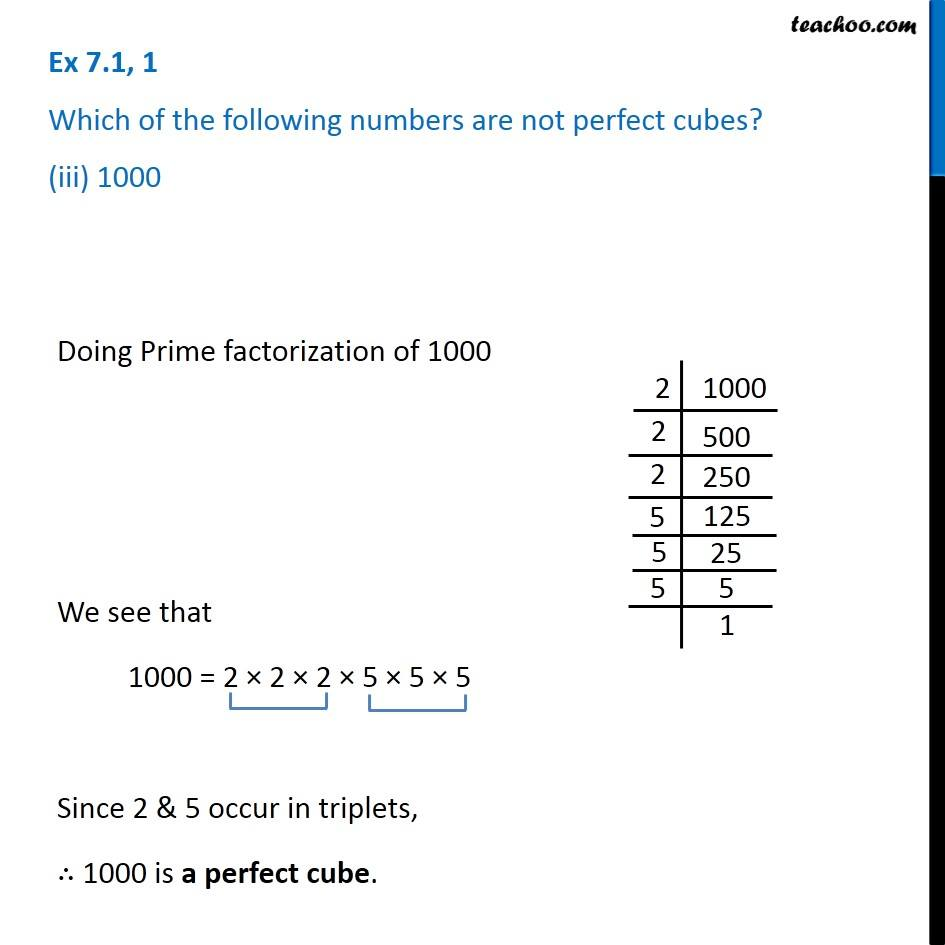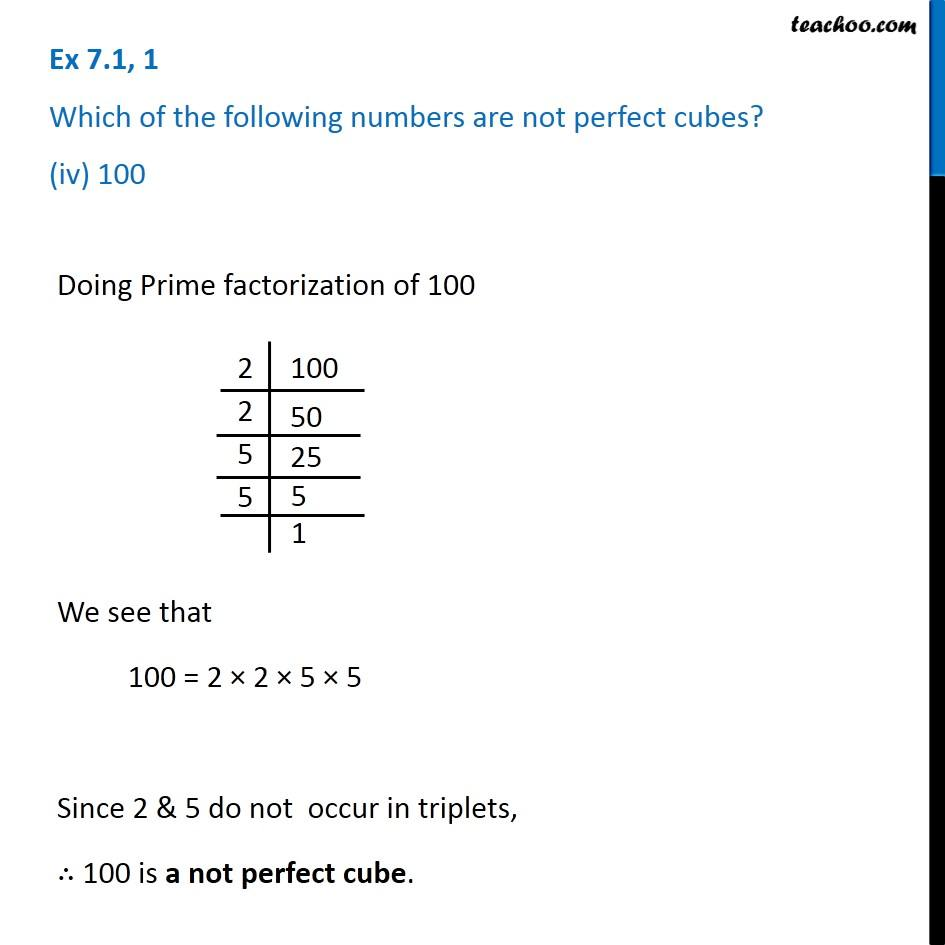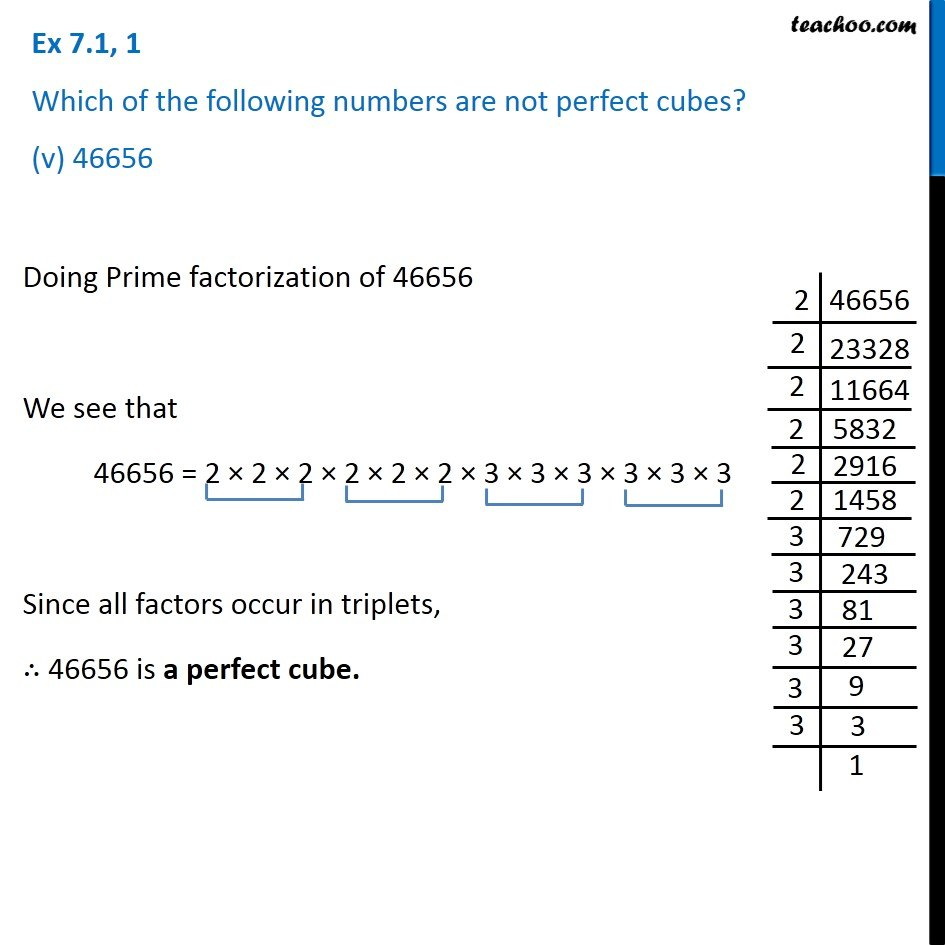Subscribe to our Youtube Channel - https://you.tube/teachoo

1. Chapter 7 Class 8 Cubes and Cube Roots
2. Serial order wise
3. Ex 7.1

Transcript

Ex 7.1, 1 Which of the following numbers are not perfect cubes? (i) 216Doing Prime factorization of 216 We see that 216 = 2 × 2 × 2 × 3 × 3 × 3 Since 2 & 3 occur in triplets, ∴ 216 is a perfect cube. Ex 7.1, 1 Which of the following numbers are not perfect cubes? (ii) 128Doing Prime factorization of 128 We see that 128 = 2 × 2 × 2 × 2 × 2 × 2 × 2 Since 2 does not occur in triplets, ∴ 128 is not a perfect cube. Doing Prime factorization of 128 We see that 128 = 2 × 2 × 2 × 2 × 2 × 2 × 2 Since 2 does not occur in triplets, ∴ 128 is not a perfect cube. Ex 7.1, 1 Which of the following numbers are not perfect cubes? (iii) 1000 Doing Prime factorization of 1000 We see that 1000 = 2 × 2 × 2 × 5 × 5 × 5 Since 2 & 5 occur in triplets, ∴ 1000 is a perfect cube. Ex 7.1, 1 Which of the following numbers are not perfect cubes? (iv) 100 Doing Prime factorization of 100 We see that 100 = 2 × 2 × 5 × 5 Since 2 & 5 do not occur in triplets, ∴ 100 is a not perfect cube. Ex 7.1, 1 Which of the following numbers are not perfect cubes? (v) 46656Doing Prime factorization of 46656 We see that 46656 = 2 × 2 × 2 × 2 × 2 × 2 × 3 × 3 × 3 × 3 × 3 × 3 Since all factors occur in triplets, ∴ 46656 is a perfect cube.

Ex 7.1